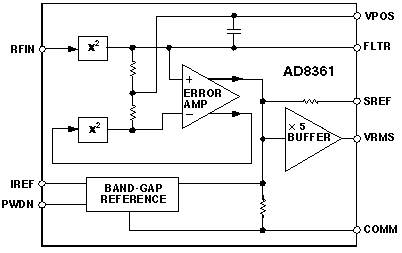# Now -True RMS-to-DC Measurements, From Low Frequencies to 2.5GHz

## Whatever the waveform, the AD8361 TruPwr™ detector IC extracts the rms value at low cost, using supply voltages from 2.7 to 5.5 V

The AD8361 is a root-mean-square (rms)-responding true-power-detector analog IC for use in high-frequency receiver and transmitter signal chains. Computing the rms value of simple and complex waveforms containing frequencies up to 2.5 GHz, it is particularly useful for measuring the rms level of high-crest-factor (peak-to-rms-ratio) signals, such as occur in systems employing ordinary and wideband code-division multiple-access (CDMA and W-CDMA). Fabricated on a proprietary high-fT silicon bipolar process, it is housed in an 8-lead µSOIC package and specified for operation from -40 to +85°C.

The AD8361 is the newest development in a line of dedicated rms-to-dc conversion integrated circuits that started in 1977 with the advent of the AD536 (Analog Dialogue 11-2). Prior to that time, large dedicated rms modules, such as Model 440, existed, but the only ICs available in that class were analog multipliers, which can be used for rms in cumbersome, expensive circuits employing squaring and division.

The AD8361 can convert a modulated RF signal with a complex waveform, containing frequencies up to 2.5 GHz, into a varying "dc" voltage representing the rms level of the signal. Highly linear and stable with temperature, it is useful for the detection of signals using CDMA, quadrature amplitude modulation (QAM), and other complex modulation schemes, with a 30-dB dynamic range. Measurement accuracy is to within 0.25 dB over a 14-dB range, and 1 dB over a 23-dB range. It requires only 4 mA from a 2.7- to 5.5-V power supply (and has a 1-µA power-down mode for conserving battery power when not in use). An evaluation board is available (AD8361-EVAL). The AD8361 is priced at \$3.75 in 1,000s.

### Why an RMS IC

RMS ICs compute the true rms value of a waveform, performing a running solution of the equation,

They produce a faithful measure of the average power in a resistive load regardless of the waveshape, with greater accuracy than devices that compute simple averages–and in a much simpler implementation at lower power and lower cost, than devices that make use of heating effects. Typical applications are found in transmitter power control and received signal strength indicators (RSSIs).

The AD8361 achieves this function through the use of a proprietary feedback technique in which the outputs of two identical squaring cells are balanced by the action of a high-gain error amplifier (Figure 1).Figure 1: Functional block diagram.

How it works: The signal to be measured is applied to the input of the first squaring cell, which presents a nominal resistance of 225 ohms between the input pin (RFIN) and COMM (connected to the ground plane). Since the input pin is at a bias voltage of about 0.8 V above ground, a coupling capacitor is required. Because this capacitor is an external component, it can be chosen to permit the measurement range to be extended to arbitrarily low frequencies.

The squaring cell generates a current proportional to VIN2 and applies it to a parallel R-C. Because of the low-pass filtering, the voltage developed across the resistor is proportional to the average value of VIN2 (and the power dissipated in the device's input resistance). Additional capacitance can be connected externally to increase the filtering time constant as desired.

This mean-square voltage is applied to one input of a high-gain error-sensing amplifier. The amplifier's other input is the output of a second squaring cell, which computes the square of the (fed-back) output of the amplifier. At equilibrium then, the amplifier's output is required to be proportional to the square root of the average value of VIN2 (i.e., the rms value of the input). A buffered version of this amplifier output becomes the device output.

The squaring cells have very wide bandwidth and are capable of responding to frequencies from dc to microwave. Because they are essentially identical and tend to track with temperature, the conversion gain is pretty much independent of their individual gains and is stable with temperature.

The AD8361 responds to inputs of up to 390 mV rms with a 3-V supply and 660 mV rms with a 5-V supply. It has a nominal conversion gain of 7.5 V/Vrms. Three operating offset modes are available, to accommodate a variety of A/D converter requirements:

• Ground-referenced mode, with zero offset,
• Internal reference mode, offsetting the output 350 mV above ground
• Supply reference mode, offsetting the output to VS/7.5.

### Ссылки

Greichen, John and Eamon Nash, "Revolutionary IC performs rms-to-dc conversion," Microwaves & RF, September, 1999, pp. 140-146. (http://www.mwrf.com)

Nonlinear Circuits Handbook, edited by D. H. Sheingold. Norwood, MA: Analog Devices, Inc., 1976.